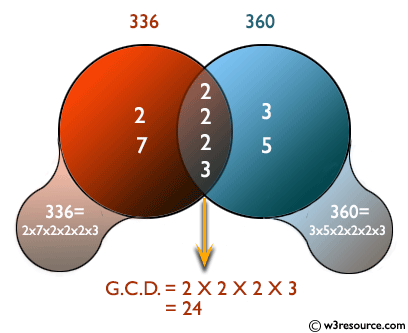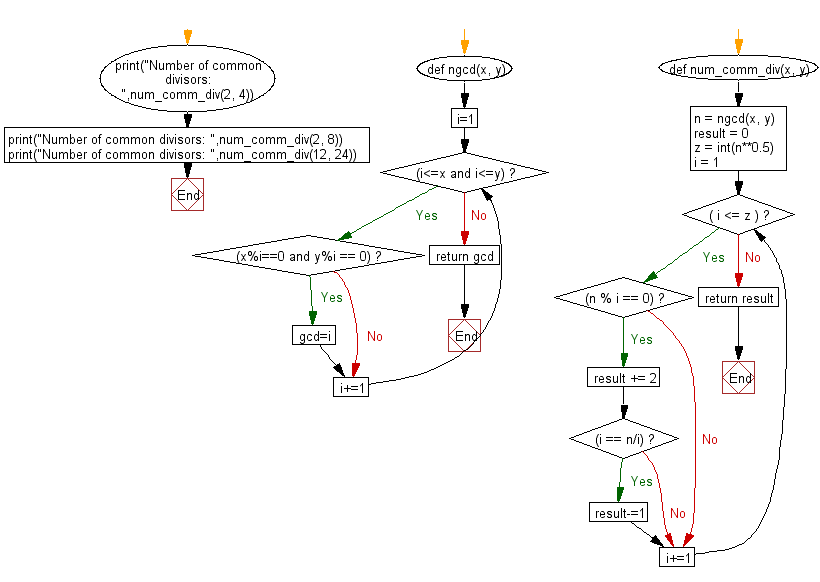﻿ Python: Find common divisors between two numbers in a given pair - w3resource# Python: Find common divisors between two numbers in a given pair

## Python Basic - 1: Exercise-29 with Solution

Write a Python program to find common divisors between two numbers in a given pair.

Pictorial Presentation:Sample Solution:

Python Code:

``````def ngcd(x, y):
i=1
while(i<=x and i<=y):
if(x%i==0 and y%i == 0):
gcd=i;
i+=1
return gcd;
def num_comm_div(x, y):
n = ngcd(x, y)
result = 0
z = int(n**0.5)
i = 1
while( i <= z ):
if(n % i == 0):
result += 2
if(i == n/i):
result-=1
i+=1
return result

print("Number of common divisors: ",num_comm_div(2, 4))
print("Number of common divisors: ",num_comm_div(2, 8))
print("Number of common divisors: ",num_comm_div(12, 24))
``````

Sample Output:

```Number of common divisors:  2
Number of common divisors:  2
Number of common divisors:  6
```

Flowchart:## Visualize Python code execution:

The following tool visualize what the computer is doing step-by-step as it executes the said program:

Python Code Editor:

Have another way to solve this solution? Contribute your code (and comments) through Disqus.

What is the difficulty level of this exercise?

Test your Programming skills with w3resource's quiz.

﻿

## Python: Tips of the Day

What is the difference between Python's list methods append and extend?

append: Appends object at the end.

```x = [1, 2, 3]
x.append([4, 5])
print (x)
```

Output:

```[1, 2, 3, [4, 5]]
```

extend: Extends list by appending elements from the iterable.

```x = [1, 2, 3]
x.extend([4, 5])
print (x)
```

Output:

```[1, 2, 3, 4, 5]
```

Ref: https://bit.ly/2AZ6ZFq